## 目标

• 定义三维向量（Vector3f）类，实现向量的常用计算方法。
• 扩展实现二维向量、四维向量。

## 实现

### 三维向量

``````public class Vector3f {
public float x, y, z;

public Vector3f() {
x = y = z = 0;
}

public Vector3f(float x, float y, float z) {
this.x = x;
this.y = y;
this.z = z;
}
}
``````

Vector3f 可以用来表示三维空间中的一个“点”，这时 (x, y, z) 描述了该“点”在自身坐标系统中的相对位置

Vector3f 也可以用来表示方向，看作是从原点 (0, 0, 0) 出发，指向 (x, y, z) 的箭头。这个“箭头”具有长度，也称为向量的模

### 零向量

3D零向量表示为 (0, 0, 0)，它是唯一一个长度为0的向量。

``````    public final static Vector3f ZERO = new Vector3f(0, 0, 0);
``````

### 负向量

``````/**
* 求负向量
*
* @return
*/
public Vector3f negate() {
return new Vector3f(-x, -y, -z);
}

/**
* 求负向量
*
* @return
*/
public Vector3f negateLocal() {
x = -x;
y = -y;
z = -z;
return this;
}
``````

• negate() 方法不会改变原向量，而是返回一个新的向量，它是原向量的负向量；
• negateLocal() 方法会改变原向量自身的值，并返回该向量本身。

### 向量大小（长度或模）

``````/**
* 返回向量的长度
*
* @return
*/
public float length() {
return (float) Math.sqrt(x * x + y * y + z * z);
}
``````

``````/**
* 返回向量长度的平方
*
* @return
*/
public float lengthSquared() {
return x * x + y * y + z * z;
}
``````

### 标量与向量的乘法

``````/**
* 标量乘法
*
* @param scalor
* @return
*/
public Vector3f mult(float scalor) {
return new Vector3f(x * scalor, y * scalor, z * scalor);
}

/**
* 标量乘法
*
* @param scalor
* @return
*/
public Vector3f multLocal(float scalor) {
x *= scalor;
y *= scalor;
z *= scalor;
return this;
}
``````

### 标准化向量

``````public final static Vector3f UNIT_X = new Vector3f(1, 0, 0);
public final static Vector3f UNIT_Y = new Vector3f(0, 1, 0);
public final static Vector3f UNIT_Z = new Vector3f(0, 0, 1);
``````

``````/**
* 求单位向量
*/
public Vector3f normalize() {
float length = x * x + y * y + z * z;
if (length != 1f && length != 0f) {
length = (float) (1.0 / Math.sqrt(length));
return new Vector3f(x * length, y * length, z * length);
}
return new Vector3f(x, y, z);
}

/**
* 求单位向量
*/
public Vector3f normalizeLocal() {
float length = x * x + y * y + z * z;
if (length != 1f && length != 0f) {
length = (float) (1.0 / Math.sqrt(length));
x *= length;
y *= length;
z *= length;
}
return this;
}
``````

### 向量的加法和减法

``````/**
* 向量加法
*
* @param v
* @return
*/
return new Vector3f(x + v.x, y + v.y, z + v.z);
}

/**
* 向量加法
* @param v
* @return
*/
x += v.x;
y += v.y;
z += v.z;
return this;
}
``````

``````/**
* 向量减法
*
* @param v
* @return
*/
public Vector3f subtract(Vector3f v) {
return new Vector3f(x - v.x, y - v.y, z - v.z);
}

/**
* 向量减法
*
* @param v
* @return
*/
public Vector3f subtractLocal(Vector3f v) {
x -= v.x;
y -= v.y;
z -= v.z;
return this;
}
``````

• 向量不能与标量或维数不同的向量相加减
• 和标量乘法一样，向量加法满足交换律，但是向量减法不满足交换律。永远有 a+b=b+a，但 a-b=-(b-a)，仅当a=b时，a-b=b-a。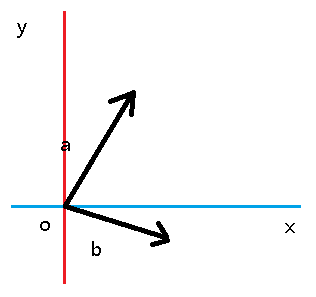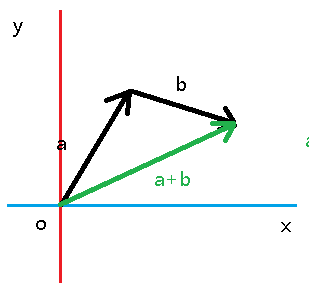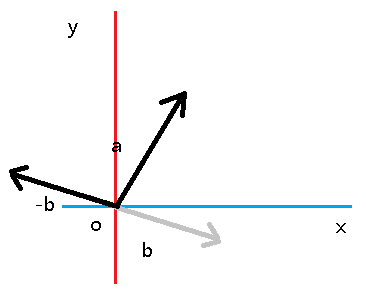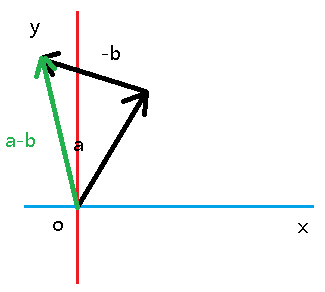### 一个点到另一个点的向量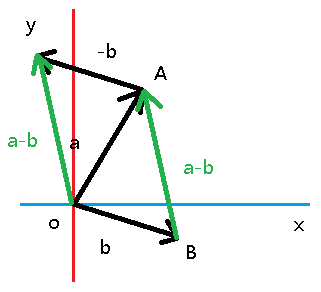### 一个点到另一个点的线性插值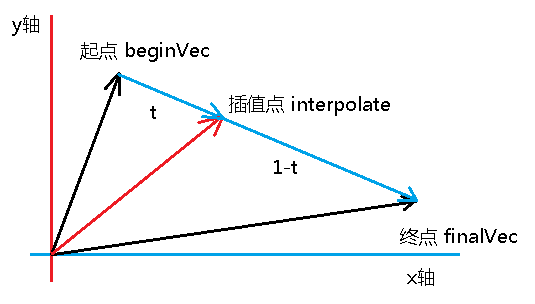``````interpolate = t * (final - begin)
``````

``````interpolate = t * final + (1 - t) * begin
``````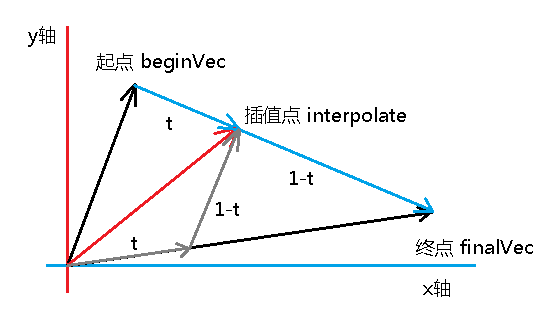``````/**
* 在当前向量与final向量之间线性插值。
*
* this=(1-changeAmnt)*this + changeAmnt * finalVec
* @param finalVec 终向量
* @param changeAmnt 插值系数，取值范围为 0.0 - 1.0。
*/
public Vector3f interpolateLocal(Vector3f finalVec, float changeAmnt) {
this.x=(1-changeAmnt)*this.x + changeAmnt*finalVec.x;
this.y=(1-changeAmnt)*this.y + changeAmnt*finalVec.y;
this.z=(1-changeAmnt)*this.z + changeAmnt*finalVec.z;
return this;
}

/**
* 在当开始向量与终向量之间线性插值。
* this=(1-changeAmnt)*beginVec + changeAmnt * finalVec
* @param beginVec 开始向量（changeAmnt = 0）
* @param finalVec 终向量（changeAmnt = 1）
* @param changeAmnt 插值系数，取值范围为 0.0 - 1.0。
*/
public Vector3f interpolateLocal(Vector3f beginVec,Vector3f finalVec, float changeAmnt) {
this.x=(1-changeAmnt)*beginVec.x + changeAmnt*finalVec.x;
this.y=(1-changeAmnt)*beginVec.y + changeAmnt*finalVec.y;
this.z=(1-changeAmnt)*beginVec.z + changeAmnt*finalVec.z;
return this;
}
``````

### 距离公式

``````/**
* 求两点之间的距离
*
* @param v
* @return
*/
public float distance(Vector3f v) {
double dx = x - v.x;
double dy = y - v.y;
double dz = z - v.z;
return (float) Math.sqrt(dx * dx + dy * dy + dz * dz);
}

/**
* 求两点之间距离的平方
*
* @param v
* @return
*/
public float distanceSquared(Vector3f v) {
double dx = x - v.x;
double dy = y - v.y;
double dz = z - v.z;
return (float) (dx * dx + dy * dy + dz * dz);
}
``````

### 向量点乘

``````/**
* 向量点乘（内积）
*
* @param v
* @return
*/
public float dot(Vector3f v) {
return x * v.x + y * v.y + z * v.z;
}
``````

``````a dot b = |a|*|b|*cos(θ)
``````

• 若 cos > 0。说明a和b的夹角 0° <= θ < 90°；
• 若 cos = 0，说明a和b正交（垂直），即 θ ≈ 90°；
• 若 cos < 0，说明a和b的夹角 90° < θ <= 180°。

### 向量之间的夹角

``````/**
* 求两个向量之间的夹角（弧度制）
* 注意：参与运算的两个向量都应该是单位向量
*
* @param v
* @return
*/
public float angleBetween(Vector3f v) {
float dotProduct = x * v.x + y * v.y + z * v.z;
float angle = (float) Math.acos(dotProduct);
return angle;
}
``````

### 向量投影

``````/**
* 向量投影
*
* @param v
* @return 返回一个新的向量，它平行于另一个向量。
*/
public Vector3f project(Vector3f v){
float n = x * v.x + y * v.y + z * v.z; // A . B
float d = v.lengthSquared(); // |B|^2
float scalor = n / d;
return new Vector3f(v.x * scalor, v.y * scalor, v.z * scalor);
}

/**
* 向量投影
* @param v
* @return 返回一个新的向量，它平行于另一个向量。
*/
public Vector3f projectLocal(Vector3f v){
float n = this.dot(v); // A . B
float d = v.lengthSquared(); // |B|^2
float scalor = n / d;
x = v.x * scalor;
y = v.y * scalor;
z = v.z * scalor;
return this;
}
``````

### 向量叉乘

``````/**
* 向量叉乘（外积）
*
* @param v
* @return 返回一个新的向量，它垂直于当前两个向量。
*/
public Vector3f cross(Vector3f v) {
float rx = y * v.z - z * v.y;
float ry = z * v.x - x * v.z;
float rz = x * v.y - y * v.x;
return new Vector3f(rx, ry, rz);
}

/**
* 向量叉乘（外积）
*
* @param v
* @return 返回一个新的向量，它垂直于当前两个向量。
*/
public Vector3f crossLocal(Vector3f v) {
float tempX = y * v.z - z * v.y;
float tempY = z * v.x - x * v.z;
z = x * v.y - y * v.x;
x = tempX;
y = tempY;
return this;
}
``````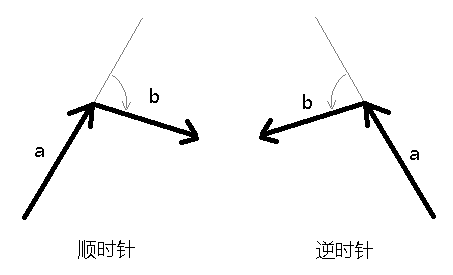### 向量的乘法

``````/**
* 向量乘法
*
* @param v
* @return
*/
public Vector3f mult(Vector3f v) {
return new Vector3f(x * v.x, y * v.y, z * v.z);
}

/**
* 向量乘法
*
* @param v
* @return
*/
public Vector3f multLocal(Vector3f v) {
x *= v.x;
y *= v.y;
z *= v.z;
return this;
}
``````

### 向量的除法

``````/**
* 标量除法
* @param scalor
* @return
*/
public Vector3f divide(float scalor) {
scalor = 1 / scalor;
return new Vector3f(x * scalor, y * scalor, z * scalor);
}

/**
* 标量除法
* @param scalor
* @return
*/
public Vector3f divideLocal(float scalor) {
scalor = 1 / scalor;
x *= scalor;
y *= scalor;
z *= scalor;
return this;
}
``````

``````/**
* 向量除法
* @param v
* @return
*/
public Vector3f divide(Vector3f v) {
return new Vector3f( x / v.x, y / v.y, z / v.z);
}

/**
* 向量除法
* @param v
* @return
*/
public Vector3f divideLocal(Vector3f v) {
x /= v.x;
y /= v.y;
z /= v.z;
return this;
}
``````

## 总结

### 二维向量叉乘

``````(x1, y1, 0) cross (x2, y2, 0) = (0, 0, x1 * y2 - y1 * x2)
``````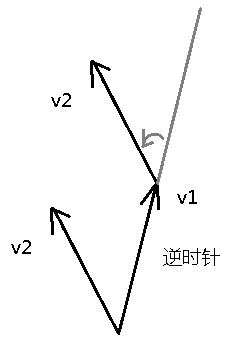``````/**
* 判别式
*/
public float determinant(Vector2f v) {
return (x * v.y) - (y * v.x);
}

public Vector3f cross(Vector2f v) {
return new Vector3f(0, 0, determinant(v));
}
``````

### 四维向量初始化

``````/**
* 使用一个三维向量+一个标量来构造四维向量。
* @param v
* @param w
*/
public Vector4f(Vector3f v, float w) {
this.x = v.x;
this.y = v.y;
this.z = v.z;
this.w = w;
}

/**
* 使用2个二维向量来构造四维向量。
* @param v0
* @param v1
*/
public Vector4f(Vector2f v0, Vector2f v1) {
this.x = v0.x;
this.y = v0.y;
this.z = v1.x;
this.w = v1.y;
}
``````